In :
import warnings
warnings.filterwarnings('ignore')
warnings.simplefilter('ignore')

In :
%matplotlib notebook
import paramonte as pm
import numpy as np
import scipy as sp
import pandas as pd
import seaborn as sns
import matplotlib as mpl
import matplotlib.pyplot as plt
print('\n'.join(f'{m.__name__} {m.__version__}' for m in globals().values() if getattr(m, '__version__', None)))
sns.set()

paramonte 2.5.2
numpy 1.20.2
scipy 1.6.1
pandas 1.2.3
seaborn 0.11.1
matplotlib 3.4.1


## Simple power-law (or linear!) regression via the ParaDRAM sampler¶

Suppose we have collected a dataset comprised of following $(x_i,y_i), \{i = 1, \ldots, 6\}$ pair observations,

\begin{eqnarray} X &=& [\, 0.5,\, 2.4,\, 3.2,\, 4.9,\, 6.5,\, 7.8\, ]\, , \\ Y &=& [\, 0.8,\, 9.3,\, 37.9,\, 68.2,\, 155,\, 198\, ]\, . \end{eqnarray}

and we would like to model the dependence of $Y$ on $X$. First thing to do in such problems is to explore the data and visualize it in search of any potential patterns, as we do below.

In :
X = np.double([0.5, 2.4, 3.2, 4.9, 6.5, 7.8])
Y = np.double([0.8, 9.3, 37.9, 68.2, 155, 198])

fig = plt.figure  ( figsize = (4.5,4)
, dpi = 200
)
ax.scatter( X
, Y
, color = "red"
, s = 5 # symbol size
)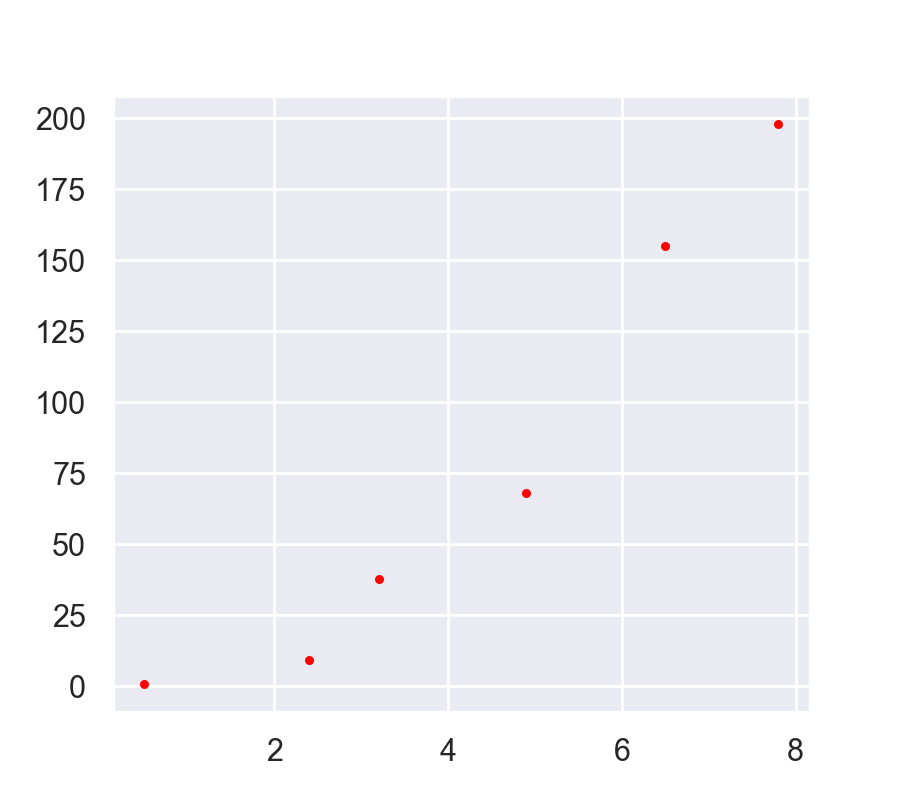Out:
<matplotlib.collections.PathCollection at 0x2d06ff57948>

Obviously, the relationship between $X$ and $Y$ in this dataset is non-linear. A fairly experienced Data Scientist could immediately guess the underlying relatioship in this dataset: power-law, which can be readily confirmed by checking the $X-Y$ relationship on a log-log plot. This works because if,

$${\large y = \alpha x^\beta \, ,}$$

then, $${\large \log(y) = \log(\alpha) + \beta \log(x) \, .}$$

In :
fig = plt.figure(figsize = (4.5,4), dpi = 200)
ax.scatter(np.log(X), np.log(Y), color = "red", s = 5)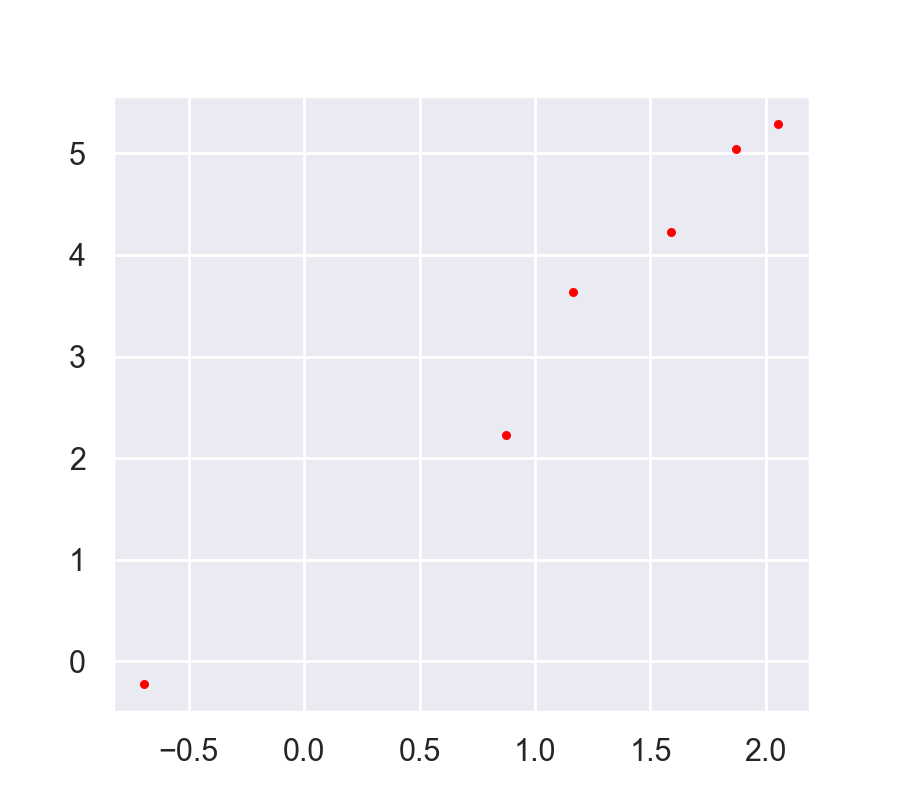Out:
<matplotlib.collections.PathCollection at 0x2d06ff60f48>

However, a simple line does not perfectly fit the (log-transformed) data either. Therefore, we must also take into account the inherent variability in our data around the linear fit.

Although not always correct, the simplest most popular assumption about the noise in data is that the $Y$ is distributed according to a Normal distribution around the best-fit line to our log-transformed data,

$${\large \pi(\log(y) \;|\; \log(x),\mu,\sigma) = \frac{1}{\sigma\sqrt{2\pi}} \exp\bigg[\;\frac{1}{2}\bigg(\frac{\log(y)\; \mu}{\sigma}\bigg)^2\bigg] } \;,$$

The unknown parameters of this Normal distribution, the mean ($\mu$) and the standard deviation ($\sigma$) would have to be then determined by fitting. Fortunately, the mean of the normal distribution can be simply taken to be the prediction of our best linear fit. Therefore, the mean is a function of the $x$ value and the slope and intercept of our linear fit to log-transformed data. However, the standard deviation would be to fit and constrained separately.

Now that we have constructed our model and the noise, we can proceed to use the principle of Maximum Likelihood to constrain the unknown parameters of the model using the Markov Chain Monte Carlo approach that is available from the ParaMonte library via the ParaDRAM() sampler class.

To summarize, the unknown parameters are the slope and intercept of the linear fit and the standard deviation (sigma) of the Normal distribution.

The probability of observing a single data point is given by the above Normal Probability Density Function (PDF). Therefore, the probability of observing all of our dataset, assuming they are independent and identically distributed (i.i.d.), is simply the multiplication of all of the individual probabilities,

\begin{eqnarray} \large \mathcal{L}(\log(Y),\log(X) \;|\; \text{intercept, slope, }\sigma) &=& \large \prod_{i=1}^{N=6}\; \pi(\log(y_i)\;|\;\log(x_i),\mu,\sigma) \; , \\ \large \mu(\log(x),\text{intercept, slope, }\sigma) &=& \large \text{intercept} + \text{slope} \times \log(x) \;, \end{eqnarray}

The likelihood is often difficult as the likelihood value is often very tiny causing underflow in computers. Therefore, we often prefer to work with the log-transformation of the likelihood which does not suffer from the same problem,

\begin{eqnarray} {\large \log\mathcal{L}(\log(Y),\log(X) \;|\; \text{intercept, slope, }\sigma) = \sum_{i=1}^{N=6} \; \log\pi(\log(y_i)\;|\;\log(x_i),\mu,\sigma) \;, } \end{eqnarray}

We now go on to implement this log-likelihood as a Python function. However, before doing so, we shall take care of an extremely important issue: The possible range of values of the parameters.

If you pay attention to the parameters, you will notice that the intercept and slope can in general be any real value from negative infinity to positive infinity. However, the third parameter, the standard deviation parameter of the Normal distribution is a strictly positive-valued number. This range-asymmetry can cause instabilities in our Markov Chain sampler.

How can we solve this and symmetrize the range of sigma? The answer is simple: We will sample $\log(\sigma)$ instead of sigma itself ($\sigma$). So our third sampling parameter will be logSigma instead of sigma, which has a symmetric possible range from negative infinity to positive infinity. Then, inside our likelihood function, we will convert logSigma back to sigma.

In :
from scipy.stats import norm

logX = np.log(X)
logY = np.log(Y)

def getLogLike(param):
"""
Return the natural logarithm of the likelihood of observing the (X,Y) dataset defined
globally outside of this function given the input parameter vector param.

Parameters
==========

param
A numpy 64-bit real vector containing the parameters of the model in the following order:
intercept, slope, log(sigma) (standard deviation of the Normal distribution).

Returns
=======
logLike
The natural logarithm of the likelihood of observing the dataset (X,Y) given param.
"""

# Compute the expected value of y, given each value of x.
# This is the mean of the Normal distribution given the x values.

mean = param + param * logX

# Compute the log-PDFs of oberserving each data point given the inpur parameters.

logProbDensities = norm.logpdf(logY, loc = mean, scale = np.exp(param)) # Note that param is logSigma.

# Compute and return the log-likliehood

return np.sum(logProbDensities)


Now let's test this likelihood function for a random input test value for the parameters to ensure it works fine. We will pick our best guess (simply by visual inspection of the data log-log plot).

In :
getLogLike(param = np.double([0.8,2,0.1]))

Out:
-6.501444916760644

We can now proceed to the set up a ParaDRAM Markov Chain Monte Carlo sampler to constrain the three unknown parameters of the model.

In :
import paramonte as pm


The following settings are optional but recommended.

In :
pmpd.spec.overwriteRequested = True # overwrite the existing output files just in case they already exist.
pmpd.spec.outputFileName = "./out/regression_powerlaw" # specify the output file prefixes.
pmpd.spec.randomSeed = 12345 # set the random seed for the sake of reproducibity.
pmpd.spec.variableNameList = ["intercept", "slope", "logSigma"] # set the output names of the parameters.
pmpd.spec.chainSize = 20000 # set the number of uniquely sampled points from the likelihood function.


Since we did not set the starting point of the MCMC sampler, the default (origin) will be used as the starting point of our search, which is not too bad. Finally, we run the sampler,

In :
pmpd.runSampler( ndim = 3 # the number of parameters
, getLogFunc = getLogLike # the objective function to sample points from.
)

ParaDRAM - NOTE: Running the ParaDRAM sampler in serial mode...
ParaDRAM - NOTE: To run the ParaDRAM sampler in parallel mode visit:
ParaDRAM - NOTE: If you are using Jupyter notebook, check the Jupyter's
ParaDRAM - NOTE: terminal window for realtime simulation progress and report.

ParaDRAM - NOTE: where you should replace pmpd with your ParaDRAM sampler's object name.



Done. We can read the generated sample to analyze and visualize the results. However, before doing so, we will make sure there is no lack of convergence in the MCMC chain by inspecting the adaptation measure of the chain that is available in the output chain file.

In :
chain = pmpd.readChain(renabled = True)

ParaDRAM - WARNING: The delimiter is neither given as input to readChain()
ParaDRAM - WARNING: nor set as a simulation specification of the ParaDRAM object.
ParaDRAM - WARNING: This information is essential, otherwise how could the output files be parsed?
ParaDRAM - WARNING: For now, the ParaDRAM sampler will assume a comma-separated
ParaDRAM - WARNING: file format for the contents of the chain file(s) to be parsed.

ParaDRAM - NOTE: 1 files detected matching the pattern: "./out/regression_powerlaw*_chain.txt"

ParaDRAM - NOTE: ndim = 3, count = 20000
ParaDRAM - NOTE: parsing file contents...
ParaDRAM - NOTE: computing the sample correlation matrix...
ParaDRAM - NOTE: creating a heatmap plot object from scratch... done in 0.016764 seconds.
ParaDRAM - NOTE: computing the sample covariance matrix...
ParaDRAM - NOTE: creating a heatmap plot object from scratch... done in 0.01007 seconds.
ParaDRAM - NOTE: computing the sample autocorrelations...
ParaDRAM - NOTE: creating a line plot object from scratch... done in 0.0 seconds.
ParaDRAM - NOTE: creating a scatter plot object from scratch... done in 0.0 seconds.
ParaDRAM - NOTE: creating a lineScatter plot object from scratch... done in 0.0 seconds.
ParaDRAM - NOTE: creating a line plot object from scratch... done in 0.0 seconds.
ParaDRAM - NOTE: creating a scatter plot object from scratch... done in 0.0 seconds.
ParaDRAM - NOTE: creating a lineScatter plot object from scratch... done in 0.0 seconds.
ParaDRAM - NOTE: creating a line3 plot object from scratch... done in 0.0 seconds.
ParaDRAM - NOTE: creating a scatter3 plot object from scratch... done in 0.0 seconds.
ParaDRAM - NOTE: creating a lineScatter3 plot object from scratch... done in 0.0 seconds.
ParaDRAM - NOTE: creating a jointplot plot object from scratch... done in 0.0 seconds.
ParaDRAM - NOTE: creating a histplot plot object from scratch... done in 0.0 seconds.
ParaDRAM - NOTE: creating a kdeplot1 plot object from scratch... done in 0.0 seconds.
ParaDRAM - NOTE: creating a kdeplot2 plot object from scratch... done in 0.0 seconds.
ParaDRAM - NOTE: creating a contour3 plot object from scratch... done in 0.0 seconds.
ParaDRAM - NOTE: creating a contourf plot object from scratch... done in 0.0 seconds.
ParaDRAM - NOTE: creating a contour plot object from scratch... done in 0.0 seconds.
ParaDRAM - NOTE: creating a grid plot object from scratch... done in 0.0 seconds.

ParaDRAM - NOTE: The processed chain files are now stored in the output variable as a
ParaDRAM - NOTE: Python list. For example, to access the contents of the first (or the only)
ParaDRAM - NOTE: chain file stored in an output variable named chainList, try:
ParaDRAM - NOTE: where you will have to replace pmpd with your ParaDRAM instance name.
ParaDRAM - NOTE: To access the plotting tools, try:
ParaDRAM - NOTE:     chainList.plot.<PRESS TAB TO SEE THE LIST OF PLOTS>
ParaDRAM - NOTE:     chainList.plot.line()         # to make 2D line plots.
ParaDRAM - NOTE:     chainList.plot.scatter()      # to make 2D scatter plots.
ParaDRAM - NOTE:     chainList.plot.lineScatter()  # to make 2D line-scatter plots.
ParaDRAM - NOTE:     chainList.plot.line3()        # to make 3D line plots.
ParaDRAM - NOTE:     chainList.plot.scatter3()     # to make 3D scatter plots.
ParaDRAM - NOTE:     chainList.plot.lineScatter3() # to make 3D line-scatter plots.
ParaDRAM - NOTE:     chainList.plot.contour()      # to make fast 2D kernel density plots.
ParaDRAM - NOTE:     chainList.plot.contourf()     # to make fast 2D kernel density filled contour plots.
ParaDRAM - NOTE:     chainList.plot.contour3()     # to make fast 3D kernel density contour plots.
ParaDRAM - NOTE:     chainList.plot.histplot()     # to make seaborn 1D distribution plots.
ParaDRAM - NOTE:     chainList.plot.kdeplot1()     # to make seaborn 1D kernel density plots.
ParaDRAM - NOTE:     chainList.plot.kdeplot2()     # to make seaborn 2D kernel density plots.
ParaDRAM - NOTE:     chainList.plot.grid()         # to make GridPlot
ParaDRAM - NOTE: To plot or inspect the variable autocorrelations or the correlation/covariance matrices, try:
ParaDRAM - NOTE:     chainList.stats.<PRESS TAB TO SEE THE LIST OF COMPONENTS>


In :
chain.plot.scatter.scatter.kws.cmap = "winter" # change the color-mapping from the default "autumn"
chain.plot.scatter( ycolumns = "AdaptationMeasure" # choose the AdaptationMeasure column of the output chain file to plot
, ccolumns = [] # set the color of the points to empty from the default logLikelihood value.
)
chain.plot.scatter.currentFig.axes.set_ylim([1.e-5,1])
chain.plot.scatter.currentFig.axes.set_yscale("log")

ParaDRAM - NOTE: making the scatter plot...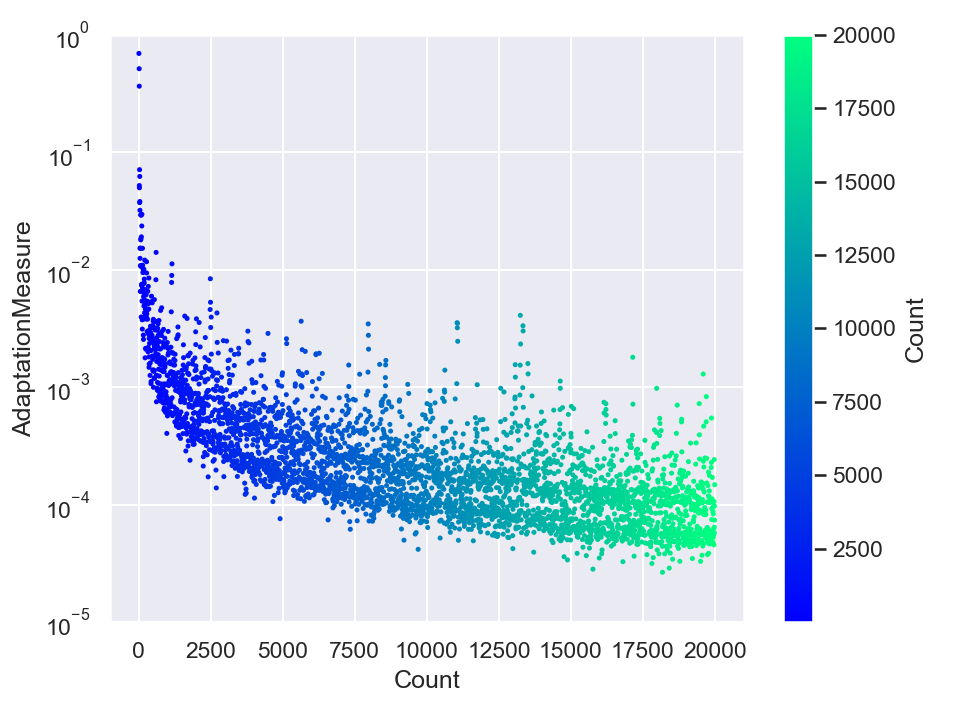done in 0.150441 seconds.


The power-law decay of the adaptation measure tells us everything has gone well with our simulation. If there were to exist any signs of a lack of convergence, we would not see this power-law decaying behavior. This power-law decay implies that the proposal adaptation decayed and diminished consistently throughout the entire simulation, as we wished.

We can now visualize the resulting refined sample by reading and plotting it from the output sample file.

In :
sample = pmpd.readSample(renabled = True)

ParaDRAM - WARNING: The delimiter is neither given as input to readSample()
ParaDRAM - WARNING: nor set as a simulation specification of the ParaDRAM object.
ParaDRAM - WARNING: This information is essential, otherwise how could the output files be parsed?
ParaDRAM - WARNING: For now, the ParaDRAM sampler will assume a comma-separated
ParaDRAM - WARNING: file format for the contents of the sample file(s) to be parsed.

ParaDRAM - NOTE: 1 files detected matching the pattern: "./out/regression_powerlaw*_sample.txt"

ParaDRAM - NOTE: ndim = 3, count = 3556
ParaDRAM - NOTE: parsing file contents...
ParaDRAM - NOTE: computing the sample correlation matrix...
ParaDRAM - NOTE: creating a heatmap plot object from scratch... done in 0.009957 seconds.
ParaDRAM - NOTE: computing the sample covariance matrix...
ParaDRAM - NOTE: creating a heatmap plot object from scratch... done in 0.01024 seconds.
ParaDRAM - NOTE: computing the sample autocorrelations...
ParaDRAM - NOTE: creating a line plot object from scratch... done in 0.0 seconds.
ParaDRAM - NOTE: creating a scatter plot object from scratch... done in 0.0 seconds.
ParaDRAM - NOTE: creating a lineScatter plot object from scratch... done in 0.0 seconds.
ParaDRAM - NOTE: creating a line plot object from scratch... done in 0.0 seconds.
ParaDRAM - NOTE: creating a scatter plot object from scratch... done in 0.0 seconds.
ParaDRAM - NOTE: creating a lineScatter plot object from scratch... done in 0.0 seconds.
ParaDRAM - NOTE: creating a line3 plot object from scratch... done in 0.0 seconds.
ParaDRAM - NOTE: creating a scatter3 plot object from scratch... done in 0.0 seconds.
ParaDRAM - NOTE: creating a lineScatter3 plot object from scratch... done in 0.0 seconds.
ParaDRAM - NOTE: creating a jointplot plot object from scratch... done in 0.0 seconds.
ParaDRAM - NOTE: creating a histplot plot object from scratch... done in 0.0 seconds.
ParaDRAM - NOTE: creating a kdeplot1 plot object from scratch... done in 0.0 seconds.
ParaDRAM - NOTE: creating a kdeplot2 plot object from scratch... done in 0.0 seconds.
ParaDRAM - NOTE: creating a contour3 plot object from scratch... done in 0.0 seconds.
ParaDRAM - NOTE: creating a contourf plot object from scratch... done in 0.0 seconds.
ParaDRAM - NOTE: creating a contour plot object from scratch... done in 0.0 seconds.
ParaDRAM - NOTE: creating a grid plot object from scratch... done in 0.0 seconds.

ParaDRAM - NOTE: The processed sample files are now stored in the output variable as a
ParaDRAM - NOTE: Python list. For example, to access the contents of the first (or the only)
ParaDRAM - NOTE: sample file stored in an output variable named sampleList, try:
ParaDRAM - NOTE: where you will have to replace pmpd with your ParaDRAM instance name.
ParaDRAM - NOTE: To access the plotting tools, try:
ParaDRAM - NOTE:     sampleList.plot.<PRESS TAB TO SEE THE LIST OF PLOTS>
ParaDRAM - NOTE:     sampleList.plot.line()         # to make 2D line plots.
ParaDRAM - NOTE:     sampleList.plot.scatter()      # to make 2D scatter plots.
ParaDRAM - NOTE:     sampleList.plot.lineScatter()  # to make 2D line-scatter plots.
ParaDRAM - NOTE:     sampleList.plot.line3()        # to make 3D line plots.
ParaDRAM - NOTE:     sampleList.plot.scatter3()     # to make 3D scatter plots.
ParaDRAM - NOTE:     sampleList.plot.lineScatter3() # to make 3D line-scatter plots.
ParaDRAM - NOTE:     sampleList.plot.contour()      # to make fast 2D kernel density plots.
ParaDRAM - NOTE:     sampleList.plot.contourf()     # to make fast 2D kernel density filled contour plots.
ParaDRAM - NOTE:     sampleList.plot.contour3()     # to make fast 3D kernel density contour plots.
ParaDRAM - NOTE:     sampleList.plot.histplot()     # to make seaborn 1D distribution plots.
ParaDRAM - NOTE:     sampleList.plot.kdeplot1()     # to make seaborn 1D kernel density plots.
ParaDRAM - NOTE:     sampleList.plot.kdeplot2()     # to make seaborn 2D kernel density plots.
ParaDRAM - NOTE:     sampleList.plot.grid()         # to make GridPlot
ParaDRAM - NOTE: To plot or inspect the variable autocorrelations or the correlation/covariance matrices, try:
ParaDRAM - NOTE:     sampleList.stats.<PRESS TAB TO SEE THE LIST OF COMPONENTS>


In :
# plot the sampled variables

for colname in sample.df.columns:
sample.plot.line.ycolumns = colname
sample.plot.line()
sample.plot.line.currentFig.axes.set_xlabel("MCMC Count")
sample.plot.line.currentFig.axes.set_ylabel(colname)
sample.plot.line.savefig( fname = "./out/traceplot_" + colname )

# plot the histograms of the sampled parameters

for colname in sample.df.columns:
sample.plot.histplot(xcolumns = colname)
sample.plot.histplot.currentFig.axes.set_xlabel(colname)
sample.plot.histplot.currentFig.axes.set_ylabel("MCMC Count")
sample.plot.histplot.savefig( fname = "./out/histogram_" + colname )

ParaDRAM - NOTE: making the line plot...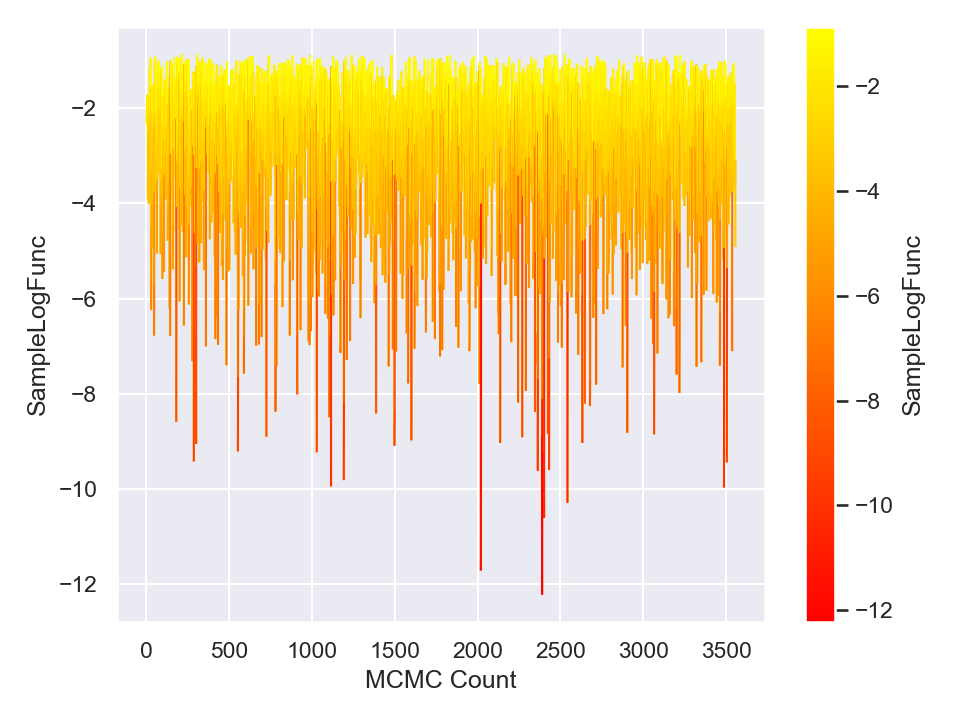done in 0.160028 seconds.
ParaDRAM - NOTE: saving the plot to file: "./out/traceplot_SampleLogFunc"
ParaDRAM - NOTE: done in 0.134692 seconds.
ParaDRAM - NOTE: making the line plot...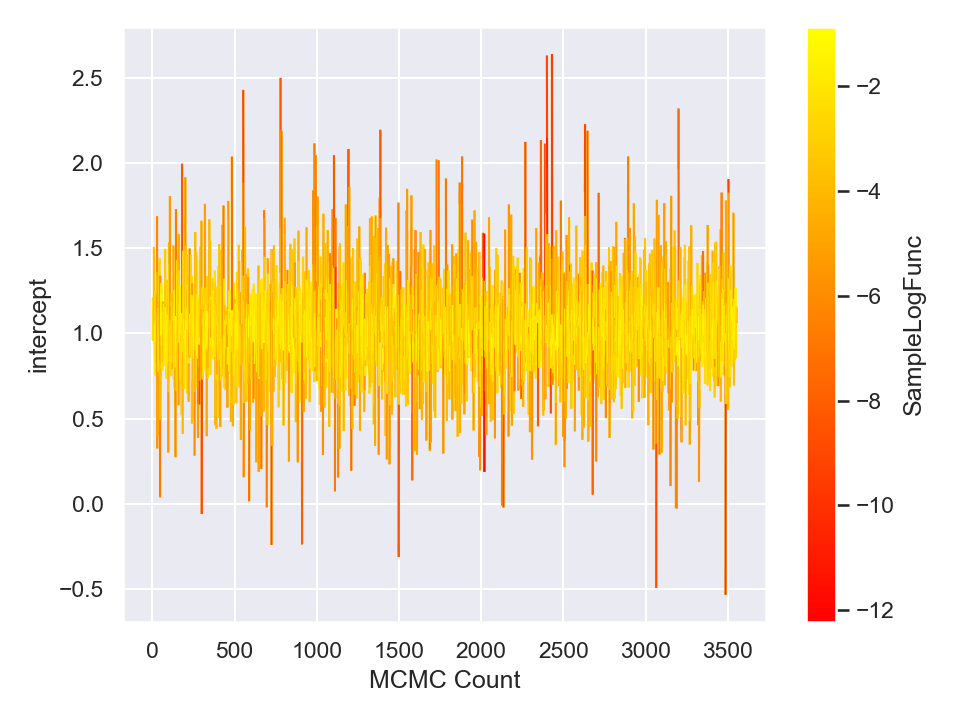done in 0.190261 seconds.
ParaDRAM - NOTE: saving the plot to file: "./out/traceplot_intercept"
ParaDRAM - NOTE: done in 0.130442 seconds.
ParaDRAM - NOTE: making the line plot...